# Difference between revisions of "Modevectors"

## Contents

### DESCRIPTION

modevectors.py is a PyMol script that was originally written to visualize results obtained from Normal Mode Analysis (NMA) by drawing arrows or vectors from a starting structure to a final structure. However, this script can be used to visualize the direction of motion between two specified states (e.g. targeted MD, comparing open and closed structures, etc). The strength of this script is that the arrows are highly customizable with numerous options to choose from (see script below). It is important to note that the two states MUST be identical except for the X, Y, and Z coordinates. That is, you cannot draw vectors between homologous structures. The default settings sets the background to white and displays the arrows along with the first object frame (in cartoon representation).

Note: Ray tracing these CGO arrows appears to be a memory intensive process so please save a session before ray tracing and use "pymol -qc" where necessary. If anybody can come up with a method to improve this please e-mail me (see address in script).

Update: A new way of drawing the arrows has been implemented for PyMOL v.1.1 and has been updated in this code (which automatically detects the version that you are using). The new method uses the built in cone primitive rather than concentric circles decreasing in size. Thus, the new method is much less memory intensive and is a significant improvement over the old method. Additionally, you may want to turn off ray shadow depending on the scene that you are viewing as shadows can be confusing to others when seen in publications.

### USAGE

load the script using the run command

```modevectors first_obj_frame, last_obj_frame [,outname=modevectors [,head=1.0 [,tail=0.3 \
[,cut=0.5 [,atom=CA [,stat=show [,factor=1.0]]]]]]]]]]]
```

Please see the script comments for further custom options. Once the script completes, it will generate a new object called "modevectors" (which can be changed through the options).

### EXAMPLES

```modevectors 1E3M, 1W7A
modevectors 1E3M, 1W7A, outname="arrows"
modevectors 1E3M, 1W7A, atom="P"
```

Copy/paste the following code to see an example of modevectors. This uses a multistate protein and the arrows are connected between the first and last states.

```# load the code
run modevectors.py
# fetch the PDBs from pdb.org
fetch 1c3y, finish=1, multiplex=0
# separate the first and last states of the NMR ensemble to individual objects
split_states 1c3y, 1, 1
split_states 1c3y, 23, 23
hide
# run the modevectors code
modevectors 1c3y_0001, 1c3y_0023
# just setup a nice representation
as cartoon, 1c3y_0001 or 1c3y_0023
show cgo, modevectors
color marine, 1c3y_0001
color purpleblue, 1c3y_0023
```

The following set of examples will illustrate the power of each optional argument. Each example should be compared to the default figure in the table below.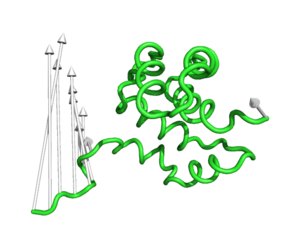Fig.1 - Default Settings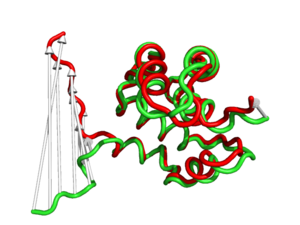Fig.2 - Arrow direction drawn from CA to CA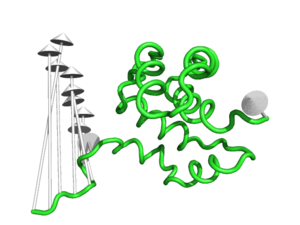Fig.3 - Arrow head radius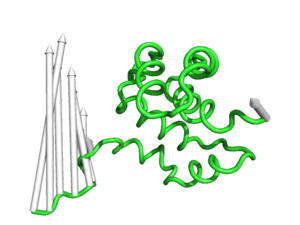Fig.4 - Arrow tail radius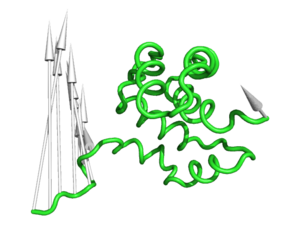Fig.5 - Arrow head length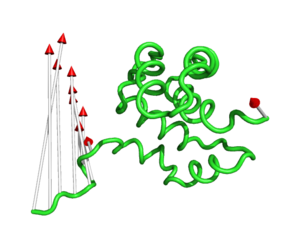Fig.6 - Arrow head RGB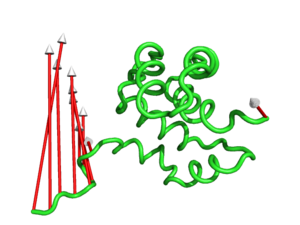Fig.7 - Arrow tail RGB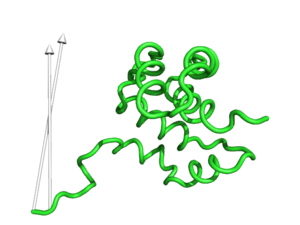Fig.8 - Arrow length cutoff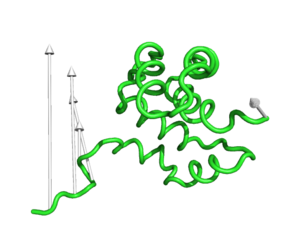Fig.9 - Skip every other arrow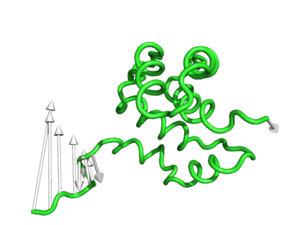Fig.10 - Subtract value from vector lengthFig.11 - Draw arrows from CB atoms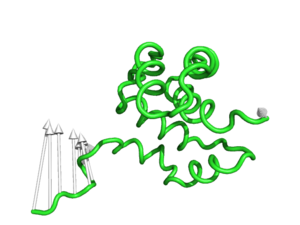Fig.12 - Shorten by 50%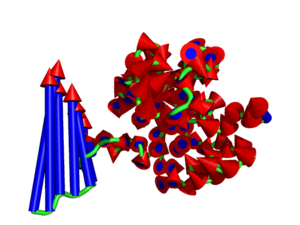Fig.13 - Multiple options being used (see final example below)
```fetch 1c3y
split_states 1c3y, 1, 1
split_states 1c3y, 23, 23
hide

#This is the default setting (Fig.1)
modevectors 1c3y_0001, 1c3y_0023

#This is shows that the direction of the arrows is drawn from the 1c3y_0001 towards 1c3y_0023 (Fig.2)
show cartoon, 1c3y_0023
color red, 1c3y_0023

#This controls the base radius of the cone/arrow head in angstroms (Fig.3)

#This controls the radius of the cylinders/arrow tails in angstroms (Fig.4)
modevectors 1c3y_0001, 1c3y_0023, tail=0.75

#This controls the length of the cone/arrow head in angstroms (Fig.5)
#Note that this option does NOT increase the vector length but simply changes the tail length

#This controls the colour of the cone/arrow head using RGB values (Fig.6)

#This controls the colour of the cylinder/arrow tails using RGB values (Fig.7)
modevectors 1c3y_0001, 1c3y_0023, tailrgb=(1.0,0.0,0.0)

#This controls the which vectors to show based on a specific cutoff value in angstroms.  Vector lengths that are less
#than the cutoff value will not be displayed (Fig.8)
modevectors 1c3y_0001, 1c3y_0023, cutoff=30.0

#This controls how many vectors to skip (integer value) and is useful when there are too many vectors showing.
#Skip=1 will show every other arrow (Fig.9)
modevectors 1c3y_0001, 1c3y_0023, skip=1

#This controls how much to cut from each vector (in angstroms).  Note that arrows will point in the opposite directions
#when too much is cutoff (resulting in a negative vector length) (Fig.10) and should be used with caution!
modevectors 1c3y_0001, 1c3y_0023, cut=15.0

#This controls which atom to draw a vector from (Fig.11).  Note that this is case-sensitive and is really only useful
#when atom=CA or when atom=P (for DNA backbones)
modevectors 1c3y_0001, 1c3y_0023, atom=CB

#This controls how much to multiple the length of each vector by (percentage increase/decrease) (Fig.12)
#This example halves the length of each vector (50%)
modevectors 1c3y_0001, 1c3y_0023, factor=0.5

#This hides the statistics which count atoms skipped, atoms counted (number of arrows showing), and number of atoms
#that did not meet the cutoff and are not shown
modevectors 1c3y_0001, 1c3y_0023, stat=hide

#Finally, this example shows multiple options being used (Fig.13)
cutoff=0.0,skip=0,cut=0,atom=CA,factor=0.8
```

### PYMOL API

```from pymol.cgo import *    # get constants
from math import *
from pymol import cmd

"""
Authors Sean Law & Srinivasa
Michigan State University
slaw_(at)_msu_dot_edu

Editor Sacha Yee

USAGE

While in PyMOL

Parameter                Preset            Type    Description
first_obj_frame          Undefined         String  Object name of the first structure.  The mode vector will propagate from this structure.  Defined by user.
last_obj_frame           Undefined         String  Object name of the last structure.  The mode vector (arrow head) will end at this structure.  Defined by user.
outname                  modevectors       String  Name of object to store mode vectors in.
tail                     0.3               Float   Radius for the cylinder of the arrow tail (cylinder)
head_length              1.5               Float   Length of the arrow head (from the base of the cone to the tip of cone)
tail_rgb                 1.0,1.0,1.0       String  RGB colour for the arrow tail.
cutoff                   4.0               Float   Skips mode vectors that do not meet the cutoff distance (in Angstroms).
skip                     0                 Integer Denotes how many atoms to skip.  No arrows will be created for skipped atoms.
cut                      0.0               Float   Truncates all arrow tail lengths (without disturbing the arrow head) (in Angstroms).
atom                     CA                String  Designates the atom to derive mode vectors from.
stat                     show              String  Keeps track and prints statistics (total modevectors, skipped, cutoff).
factor                   1.0               Float   Multiplies each mode vector length by a specified factor.
Values between 0 and 1 will decrease the relative mode vector length.
Values greater than 1 will increase the relative mode vector length.

"""

framefirst=cmd.get_model(first_obj_frame)
framelast=cmd.get_model(last_obj_frame)
objectname=outname
factor=float(factor)
cutoff=float(cutoff)
skip=int(skip)
cut=float(cut)
atomtype=atom.strip('"[]()')

tailrgb=tailrgb.strip('" []()')
tr,tg,tb=map(float,tailrgb.split(','))

version=cmd.get_version()
pi=4*atan2(1,1)
arrow=[]
arrowtail = []
x1 = []
y1 = []
z1 = []
x2 = []
y2 = []
z2 = []
exit_flag=0

##############################################################
#                                                            #
# Define an object called "tail" and store the tail and  a   #
# circular base of the triangle in this object.              #
#                                                            #
##############################################################

skipcount=0
skipcounter=0
keepcounter=0
atom_lookup={}
for atom in framefirst.atom:
if atom.name == atomtype:
if skipcount == skip:
x1.append(atom.coord)
y1.append(atom.coord)
z1.append(atom.coord)

##########################################
#                                        #
# Set atom_lookup for a specific atom    #
# equal to ONE for the first input set.  #
# This dictionary will be used as a      #
# reference for the second set.          #
#                                        #
##########################################

current_atom="CHAIN "+atom.chain+" RESID "\
+atom.resi+" RESTYPE "\
+atom.resn+\
" ATMNUM "+str(atom.index)
#				print current_atom
atom_lookup['current_atom']=1

skipcount=0
keepcounter=keepcounter+1
else:
#				print skipcount
skipcount=skipcount+1
skipcounter=skipcounter+1

skipcount=0
for atom in framelast.atom:
if atom.name == atomtype:
if skipcount == skip:
x2.append(atom.coord)
y2.append(atom.coord)
z2.append(atom.coord)

#########################################
#                                       #
# Get atom information from second set  #
# and compare with first set.  All      #
# atoms from this second set MUST be    #
# found in the first set!  Otherwise,   #
# the script will exit with an error    #
# since modevectors can only be created #
# by calculating values from identical  #
# sources.                              #
#                                       #
#########################################

current_atom="CHAIN "+atom.chain+" RESID "\
+atom.resi+" RESTYPE "\
+atom.resn+\
" ATMNUM "+str(atom.index)
#				print current_atom
if atom_lookup.has_key('current_atom') != 1:
print "\nError: "+current_atom+" from \""\
+last_obj_frame+\
exit_flag=1
break

skipcount=0
else:
skipcount=skipcount+1

if exit_flag == 1:
###########################################
#                                         #
# Exit script because an atom cannot be   #
# found in both input files               #
#                                         #
###########################################
return

cutoff_counter=0 # Track number of atoms failing to meet the cutoff

###################################################
#                                                 #
# Check that the two selections/PDB files contain #
# the same number of atoms.                       #
#                                                 #
###################################################

if len(x2) != len(x1):
print "\nError: \""+first_obj_frame+\
"\" and \""+last_obj_frame+\
"\" contain different number of residue/atoms."
return
else:
#Continue with representing modevectors!
#########################################
#                                       #
# Delete old selection or object if it  #
# exists so that it can be overwritten  #
#                                       #
#########################################
save_view=cmd.get_view(output=1,quiet=1)
cmd.delete(name=outname)
cmd.hide(representation="everything",selection=first_obj_frame)
cmd.hide(representation="everything",selection=last_obj_frame)

###################################################
#                                                 #
# Begin drawing arrow tails                       #
#                                                 #
###################################################

arrowtail = []
for mv in range(len(x1)):
vectorx=x2[mv]-x1[mv]
vectory=y2[mv]-y1[mv]
vectorz=z2[mv]-z1[mv]
length=sqrt(vectorx**2+vectory**2+vectorz**2)
if length < cutoff:
cutoff_counter=cutoff_counter+1
continue
t=1.0-(cut/length)
x2[mv]=x1[mv]+factor*t*vectorx
y2[mv]=y1[mv]+factor*t*vectory
z2[mv]=z1[mv]+factor*t*vectorz
vectorx=x2[mv]-x1[mv]
vectory=y2[mv]-y1[mv]
vectorz=z2[mv]-z1[mv]
length=sqrt(vectorx**2+vectory**2+vectorz**2)
d=arrow_head_length # Distance from arrow tip to arrow base
t=1.0-(d/length)
tail = [
# Tail of cylinder
CYLINDER, x1[mv],y1[mv],z1[mv]\
,x1[mv]+(t+0.01)*vectorx,y1[mv]+(t+0.01)*vectory,z1[mv]+(t+0.01)*vectorz\
]
arrow.extend(tail)

x=x1[mv]+t*vectorx
y=y1[mv]+t*vectory
z=z1[mv]+t*vectorz
dx=x2[mv]-x
dy=y2[mv]-y
dz=z2[mv]-z
seg=d/100
intfactor=int(factor)
if (version < 1.1): #Version >= 1.1 has cone primitive
for i in range (100,0,-1): #i=100 is tip of cone
print i
t1=seg*i
t2=seg*(i+1)
CYLINDER, x+t2*dx,y+t2*dy,z+t2*dz\
,x+t1*dx,y+t1*dy,z+t1*dz\
]
else:
CONE, x, y ,z, x+d*dx, y+d*dy, z+d*dz, arrow_head_radius, 0.0, hr, hg, hb, hr, hg, hb, 1.0, 1.0 ]

##############################################################
#                                                            #
# Load the entire object into PyMOL                          #
#                                                            #
# Print statistics if requested by user                      #
#                                                            #
##############################################################

if stat == "show":
natoms=skipcounter+keepcounter
print "\nTotal number of atoms = "+str(natoms)
print "Atoms skipped = "+str(skipcounter)
if keepcounter-cutoff_counter > 0:
print "Atoms counted = "+str(keepcounter-cutoff_counter)+" (see PyMOL object \""+outname+"\")"
else:
print "Atoms counted = "+str(keepcounter-cutoff_counter)+" (Empty CGO object not loaded)"
print "Atoms cutoff  = "+str(cutoff_counter) #Note that cutoff occurs AFTER skipping!
if keepcounter-cutoff_counter > 0:
cmd.load_cgo(arrow,objectname) #Ray tracing an empty object will cause a segmentation fault.  No arrows = Do not display in PyMOL!!!
cmd.show(representation="cartoon",selection=first_obj_frame)
cmd.cartoon("tube")
cmd.show(representation="cartoon",selection=last_obj_frame)
cmd.hide(representation="cartoon",selection=last_obj_frame)
cmd.bg_color(color="white")
cmd.set_view(save_view)
return

cmd.extend("modevectors",modevectors)
```